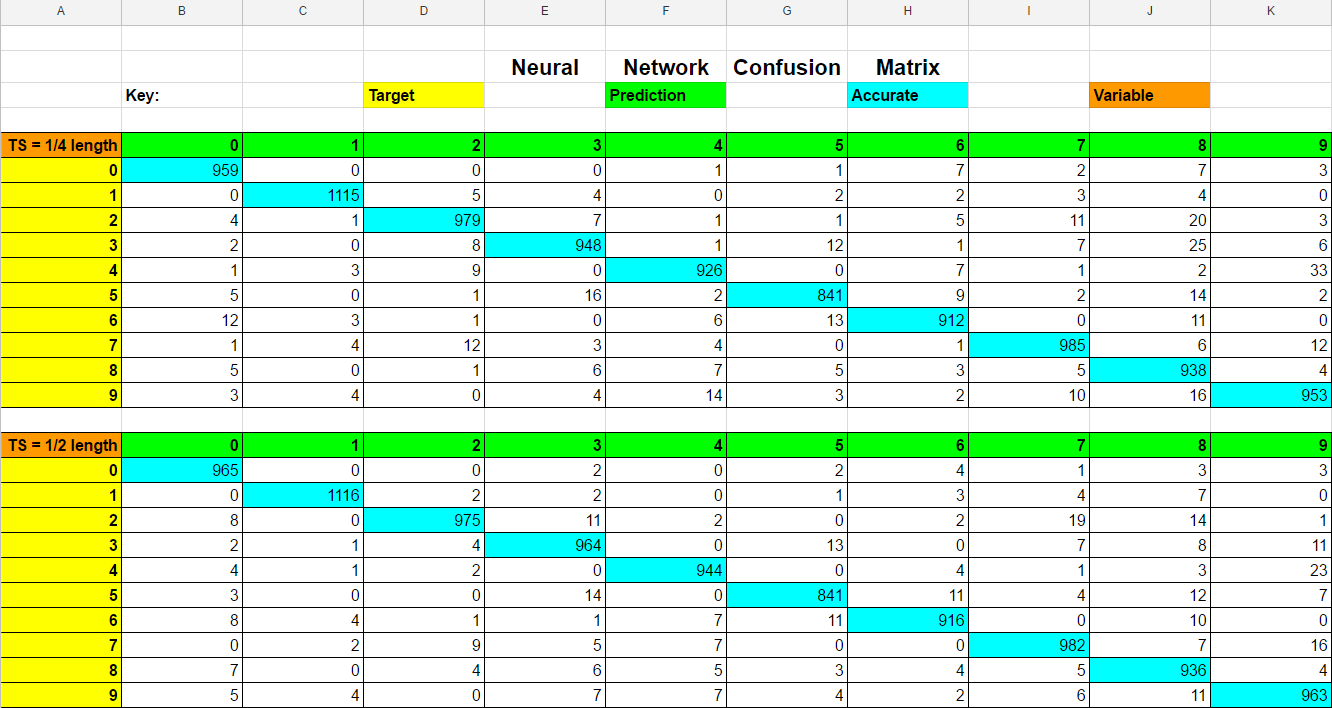# Confusion Matrix

## What is a confusion matrix?

In machine learning, a confusion matrix is an nxn matrix such that each row represents the true classification of a given piece of data and each column represents the predicted classification (or vise versa). By looking at a confusion matrix, one can determine the accuracy of the model by looking at the values on the diagonal to determine the number of correct classifications - a good model will have high values along the diagonal and low values off the diagonal. Further, one can tell where the model is struggling by assessing the highest values not on the diagonal.  Together, these analyses are useful to identify cases where the accuracy may be high but the model is consistently misclassifying the same data.

## Example Confusion Matrix

Here is an example of a confusion matrix created by a neural network analyzing the MNIST dataset. As a reminder, the MNIST dataset is a dataset consisting of handwritten digits ranging from 0-9. The neural network analyzing the MNIST dataset looks at an image and determines what the digit in the image is.Some things we can conclude given the confusion matrix above:

1. The network has a fairly high level of accuracy due to the large numbers on the diagonal and smaller numbers everywhere else.

2. The network struggles classifying the number 5 and often confuses it with the numbers 3,6, and 8.

3. Although the network performs well on the number 4, it has a serious problem misclassifying it with the number 9, as seen with the 33 misclassifications in the matrix.

4. The network does best classifying the number 1.

In practice, we take a look at the parameters in our network alongside the misclassified data and tune the parameters to improve the overall performance of the network.